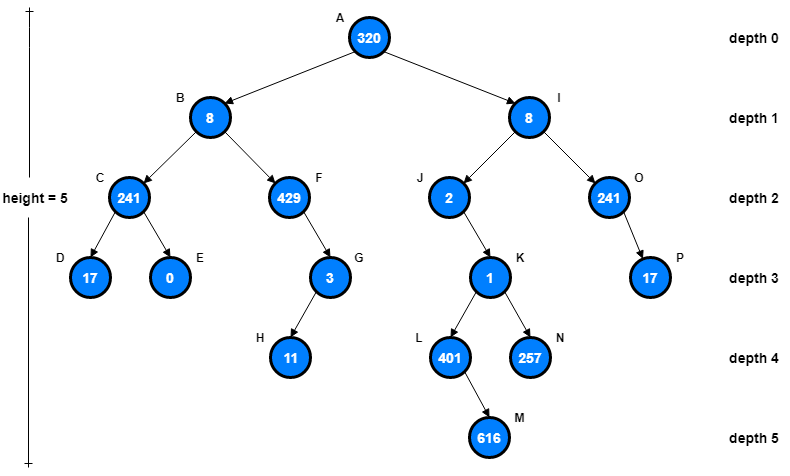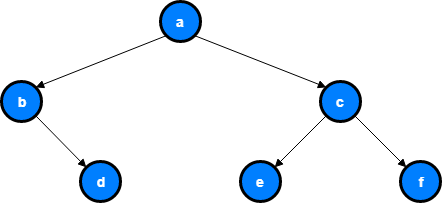# Binary Tree

Binary Tree is a classical data structure in Computer Science. It is a non-linear data structure and formally a binary tree is either empty or a root node with a left binary tree and a right binary tree.

The left binary tree is sometimes referred to as the left subtree of the root and the right binary tree is referred to as the right subtree of the root.

Binary trees most commonly occur in the context of Binary Search Trees (BST), where keys are stored in a sorted fashion but in this article we will only focus on Binary Trees. Binary Search Trees (BST) will be explained deeply in another article.In this graphical representation of Binary Tree, Node A is the root and Node B and I are the left and right children of root A.

The depth of a node n is the number of nodes on the search path from the root to n, not including n itself. The height of a binary tree is the maximum depth of any node in that tree. A level of a tree is all nodes at the same depth.

## Implementation of Binary Tree

Just like we did in Linked List, we need to start the implementation by defining a root Node and its left and right children.

``````class BinaryTree:
def __init__(self, data):
self.left = None
self.right = None
self.root = data
``````

Notice that constructor function expects some kind of data to store in the root. If we want to expand our tree beyond the root node, we will need funcions to insert new data to left and right nodes.

### insert_left function

Let’s implement `insert_left()` function together. We must consider two possibilities to be able to implement this function. First case is a node with no existing left child and the second case is node with an existing left child.

``````def insert_left(self, value):
if self.left is None:
self.left = BinaryTree(value)
else:
new_node = BinaryTree(value)
new_node.left = self.left
self.left = new_node
``````

When there is no left child, all we need to do is simply create a left child with creating a new node. In the second case, where there is already an existing left child, we are inserting as new node and pushing existing child node one level down in the tree.

### insert_right function

Implementation of `insert_right()` function is symmetric with the implementation of `insert_left()` function.

``````def insert_right(self, value):
if self.right is None:
self.right = BinaryTree(value)
else:
new_node = BinaryTree(value)
new_node.right = self.right
self.right = new_node
``````

There will be either no right child or there will be an existing right child which will be pushed down one level with the addition of new right child.

## Implementation Summary

let’s summarize what we implemented so far by adding getter and setter functions to call existing nodes.

``````class BinaryTree:
def __init__(self, data):
self.left = None
self.right = None
self.root = data

def insert_left(self, value):
if self.left is None:
self.left = BinaryTree(value)
else:
new_node = BinaryTree(value)
new_node.left = self.left
self.left = new_node

def insert_right(self, value):
if self.right is None:
self.right = BinaryTree(value)
else:
new_node = BinaryTree(value)
new_node.right = self.right
self.right = new_node

def get_right_child(self):
return self.right

def get_left_child(self):
return self.left

def set_root_value(self, value):
self.root = value

def get_root_value(self):
return self.root
``````

## Binary Tree Traversals

Traversing is the task of visiting all nodes of the tree at least once. The tree is not a linear data structure, hence there are many ways of traversing it.

The three most commonly used traversing methods are; pre-order, in-order and post-order.### Pre-order Traversal

In this traversal mode, one starts from the root, move to left child, then right child.

So in our case, order will be: a, b, d, c, e, f

``````def pre_order(self):
print(self.value)

if self.left_child:
self.left_child.pre_order()

if self.right_child:
self.right_child.pre_order()
``````

### In-order Traversal

In this traversal mode, one starts visiting with the left child, followed by its parent and then the right child.

So in our case, order will be: b, d, a, e, c, f

``````def in_order(self):
if self.left:
self.left.in_order()

print(self.root)

if self.right:
self.right.in_order()
``````

### Post-order Traversal

In this traversal mode, one starts from the left child, move to the right child, and terminate at the root.

So in our case, order will be: d, b, e, f, c, a

``````def post_order(self):
if self.left:
self.left.post_order()

if self.right:
self.right.post_order()

print(self.root)
``````

## Example

To test our code, we can try to implement the example tree in the traversals.

So;

• node a is our root node.
• node b is the left child of node a
• node c is the right child of node a
• node d is the right child of node b
• node e is the left child of node c
• node f is the right child of node c

if we want to implement this structure in our code, it will look like this;

``````a_node = BinaryTree('a')
a_node.insert_left('b')
a_node.insert_right('c')

b_node = a_node.left
b_node.insert_right('d')

c_node = a_node.right
c_node.insert_left('e')
c_node.insert_right('f')

d_node = b_node.right
e_node = c_node.left
f_node = c_node.right

print(a_node.root)  # a
print(b_node.root)  # b
print(c_node.root)  # c
print(d_node.root)  # d
print(e_node.root)  # e
print(f_node.root)  # f

print(a_node.pre_order())  # abdcef
print(a_node.in_order())  # bdaecf
print(a_node.post_order())  # dbefca
``````

## Summary

Binary Tree is a fundamental data structure in computer science and this article is just scratching the surface. I will continue writing more about trees in new articles about searching (Depth-First Search - DFS, Breadth-First Search - BFS), Binary Search Trees (BST), balanced trees, AVL trees, tree treversal etc.

1. Wikipedia, Binary Tree